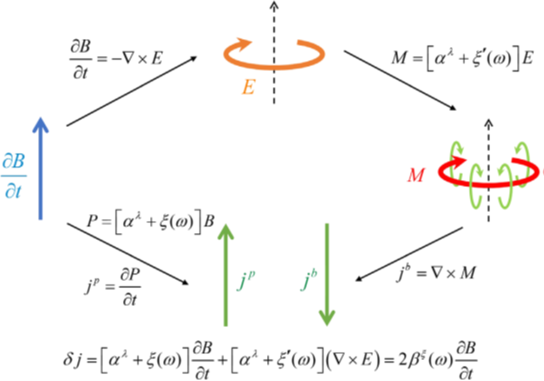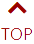﻿ 谢心澄课题组及合作者在反对称磁电耦合相关研究中取得新进展-北京大学物理学院供稿：王茂原  |   编校：孙祎、孙嘉琪   |   编辑：孙嘉琪   |   审核：冯济

jp = t P = ( t α )B + α( t B )

jb = × M = ( × α ) E + α( × E )

j = jp + jb = ( t α )B + ( × α ) E

P = (α + β) B

M = (α – β) E

j = jp + jb = t [ α + β(ω) ]B + { × [ α - β(ω) ] } ⋅ E + 2β(ω)t B2022年6月10日，相关研究成果以“新型含时反对称磁电耦合”（New Type of Anticommutative Dynamical Magnetoelectric Response）为题在线发表于《物理评论快报》（Physical Review Letters）。王茂原为第一作者与通讯作者，刘海文和谢心澄为共同作者。